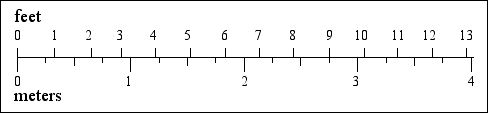## Conversion Of Meters To Feet And Inches Using A Mathematical Formula.

Asked By 0 points N/A Posted on -

Hi. I am not from a mathematical background. I want to know the formula to convert meters to feet and inches, but I am confused between the values. Please help. Thanks!SHARE
Answered By 0 points N/A #295011

## Conversion Of Meters To Feet And Inches Using A Mathematical Formula.

Hi. A meter is an SI unit of distance and length. SI symbol for a meter is “m.” An inch is a unit of measurement. Twelve inches is equal one foot. A foot is a unit for measuring length in different systems. The symbol is ft. Conversion of meters to feet and inches is as follow: 1 meter is equal to 3.28084 ft.For example, 17 meters = 3.28084*17 which is equals to 55.77428 ft . Now 1 meter is equal to 39.370078 inches. For example, 0.7 meters = 39.370078*0.7 which are equals to 27.5590546 inches.